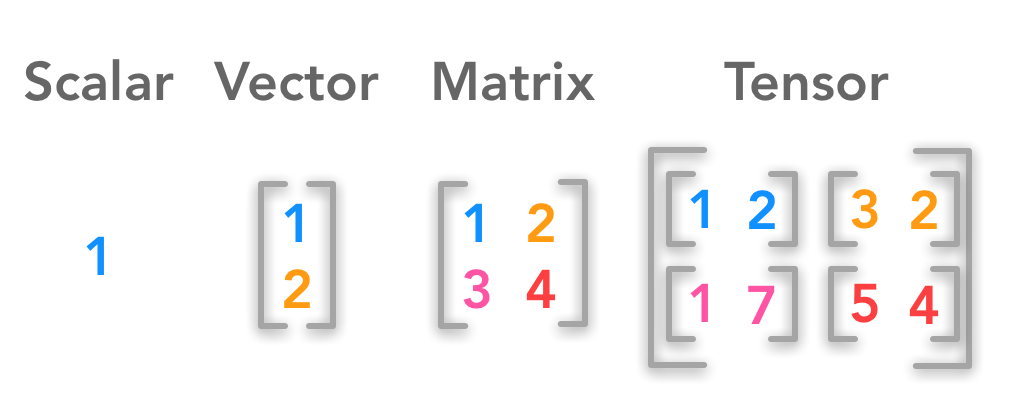﻿ 标量、向量、矩阵、张量之间的区别和联系 – 蒋智昊的博客# 标量、向量、矩阵、张量之间的区别和联系1、标量

`x = 1`

2、向量

```import numpy as np
# 行向量
a = np.array([1,2,3,4])```

3、矩阵

```import numpy as np
# 矩阵
a = np.array([[1,2,3], [4,5,6], [7,8,9]])```

4、张量

```import numpy as np
# 张量
a = np.array([[[1,2],[3,4]],[[5,6],[7,8]]])```